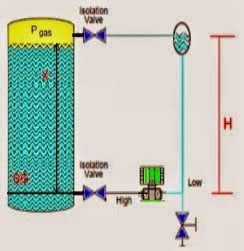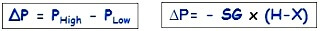# Closed tank DP Level Transmitter with wet leg Zero elevation Calibration

Schematic Diagram of a Closed tank DP Level Transmitter with wet leg Zero elevation CalibrationClosed tank DP Level Transmitter with wet leg Zero elevation CalibrationFormulas:

Min range = – SG . H

Max range = – SG . (H-X)

Example:

If the height of an X is 2 meters and height H is 2.5 meters, there is fluid in the tank which has a density of 1 g / cm3, what is the range of calibration on the Trasmitter?

SG = 1

Min range = – 1 x (2.5 – 0)

= – 1 x 2,5 m
= – 2.5 mH 2 O

Max range = -1 X (2.5 – 2)

= -1 X 0.5 m
= -0.5 MH 2 O

The Transmitter Range = -2.5 to -0.5 mH 2 O

Be the first to get exclusive content straight to your email.
We promise not to spam you. You can unsubscribe at any time.

### 3 thoughts on “Closed tank DP Level Transmitter with wet leg Zero elevation Calibration”

1.Thanks for sharing the knowledge.

2.Dear Sir

We need to have calculation to calculate the Differential Level in mm of H2o to exact level in Cum for Gas Application

We have Cryogenic Tank filled with Nitrogen Gas @ -187 deg cent.

We are putting differential gauge which is giving reading in mm of H20. we need to convert the same in to cum of gas

3.